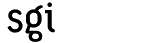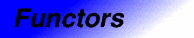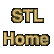# mem_fun_t<Result, X>Categories: functors, adaptors Component type: type

### Description

Mem_fun_t is an adaptor for member functions. If X is some class with a member function Result X::f() (that is, a member function that takes no arguments and that returns a value of type Result ), then a mem_fun_t<Result, X> is a function object adaptor that makes it possible to call f() as if it were an ordinary function instead of a member function.

Mem_fun_t<Result, X>'s constructor takes a pointer to one of X's member functions. Then, like all function objects, mem_fun_t has an operator() that allows the mem_fun_t to be invoked with ordinary function call syntax. In this case, mem_fun_t's operator() takes an argument of type X*.

If F is a mem_fun_t that was constructed to use the member function X::f, and if x is a pointer of type X*, then the expression F(x) is equivalent to the expression x->f(). The difference is simply that F can be passed to STL algorithms whose arguments must be function objects.

Mem_fun_t is one of a family of member function adaptors. These adaptors are useful if you want to combine generic programming with inheritance and polymorphism, since, in C++, polymorphism involves calling member functions through pointers or references.

As with many other adaptors, it is usually inconvenient to use mem_fun_t's constructor directly. It is usually better to use the helper function mem_fun instead.

### Example

```struct B {
virtual void print() = 0;
};

struct D1 : public B {
void print() { cout << "I'm a D1" << endl; }
};

struct D2 : public B {
void print() { cout << "I'm a D2" << endl; }
};

int main()
{
vector<B*> V;

V.push_back(new D1);
V.push_back(new D2);
V.push_back(new D2);
V.push_back(new D1);

for_each(V.begin(), V.end(), mem_fun(&B::print));
}
```

### Definition

Defined in the standard header functional, and in the nonstandard backward-compatibility header function.h.

### Template parameters

Parameter Description Default
Result The member function's return type.
X The class whose member function the mem_fun_t invokes.

### Type requirements

• X has at least one member function that takes no arguments and that returns a value of type Result. 

### Public base classes

unary_function<X*, Result>

### Members

Member Where defined Description
argument_type Adaptable Unary Function The type of the argument: X*
result_type Adaptable Unary Function The type of the result: Result
```Result operator()(X* x) const
```
Unary Function Function call operator. Invokes x->f(), where f is the member function that was passed to the constructor.
```explicit mem_fun_t(Result (X::*f)())
```
mem_fun_t See below.
```template <class Result, class X>
mem_fun_t<Result, X>
mem_fun(Result (X::*f)());
```
mem_fun_t See below.

### New members

These members are not defined in the Adaptable Unary Function requirements, but are specific to mem_fun_t.
Member Description
```explicit mem_fun_t(Result (X::*f)())
```
The constructor. Creates a mem_fun_t that calls the member function f.
```template <class Result, class X>
mem_fun_t<Result, X>
mem_fun(Result (X::*f)());
```
If f if of type Result (X::*) then mem_fun(f) is the same as mem_fun_t<Result, X>(f), but is more convenient. This is a global function, not a member function.

### Notes

 The type Result is permitted to be void. That is, this adaptor may be used for functions that return no value. However, this presents implementation difficulties. According to the draft C++ standard, it is possible to return from a void function by writing return void instead of just return. At present, however (early 1998), very few compilers support that feature. As a substitute, then, mem_fun_t uses partial specialization to support void member functions. If your compiler has not implemented partial specialization, then you will not be able to use mem_fun_t with member functions whose return type is void.